# Hidden Markov Models: The Viterbi Algorithm

I just finished working on LEARNINGlover.com: Hidden Marokv Models: The Viterbi Algorithm. Here is an introduction to the script.

Suppose you are at a table at a casino and notice that things don’t look quite right. Either the casino is extremely lucky, or things should have averaged out more than they have. You view this as a pattern recognition problem and would like to understand the number of ‘loaded’ dice that the casino is using and how these dice are loaded. To accomplish this you set up a number of Hidden Markov Models, where the loaded die are the latent variables, and would like to determine which of these, if any is more likely to be using.

First lets go over a few things.

We will call each roll of the dice an observation. The observations will be stored in variables o1, o2, …, oT, where T is the number of total observations.

To generate a hidden Markov Model (HMM)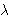we need to determine 5 parameters:

• The N states of the model, defined by S = {S1, …, SN}
• The M possible output symbols, defined by= {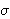1,2, …,M}
• The State transition probability distribution A = {aij}, where aij is the probability that the state at time t+1 is Sj, given that the state at time t is Si.
• The Observation symbol probability distribution B = {bj(k)} where bj(k) is the probability that the symbolk is emitted in state Sj.
• The initial state distribution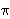= {i}, wherei is the probability that the model is in state Si at time t = 0.

The HMMs we’ve generated are based on two questions. For each question, you have provided 3 different answers which leads to 9 possible HMMs. Each of these models has its corresponding state transition and emission distributions.

• How often does the casino change dice?
• 0) Dealer Repeatedly Uses Same Dice
• 1) Dealer Uniformly Changes Die
• 2) Dealer Rarely Uses Same Dice
• Which sides on the loaded dice are more likely?
• 0) Larger Numbers Are More Likely
• 1) All Numbers Are Equally Likely
• 2) Smaller Numbers Are More Likely
How often does the casino change dice?
Which sides on
are more likely?
 (0, 0) (0, 1) (0, 2) (1, 0) (1, 1) (1, 2) (2, 0) (2, 1) (2, 2)

One of the interesting problems associated with Hidden Markov Models is called the Decoding Problem, which asks the question “What is the most likely sequence of states that the HMMwould go through to generate the sequence O = o1, o2, …, oT?

The Viterbi algorithm finds answers this question using Dynamic Programming. It creates an auxiliary variable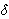t(i) which has the highest probability that the partial observation sequence o1, …, ot can have, given that the current state is i. This variable can be calculated by the following formula:t(i) = maxq1, …, qt-1 p{q1, …, qt-1, qt = i, o1, …, ot |}.1(j) =jbj(o1), for 1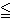jN.

Once we have calculatedt(j) we also keep a pointer to the max state. We can then find the optimal path by looking at arg max 1jNT(j) and then backtrack the sequence of states using the pointer.

There is more on this example at LEARNINGlover.com: Hidden Marokv Models: The Viterbi Algorithm.

Some further reading on Hidden Markov Models: